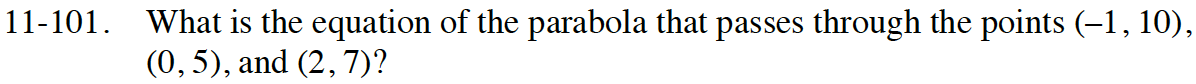Home > INT3 > Chapter 11 > Lesson 11.2.4 > Problem11-101

11-101.

What is the equation of the parabola that passes through the points (–1, 10), (0, 5), and (2, 7)?11-101 HW eTool (Desmos). Desmos Accessibility Homework Help ✎Create a system of three equations in three variables using the points and the equation y = ax2 + bx + c.

a − b + c = 10
c = 5
4a + 2b + c = 7

Substitute the value for c into the first and third equations to simplify.
You now have a system of two equations with two variables to solve.

y = 2x2 − 3x + 5

Use the eTool below to check your solution graphically.
Click the link at right for the full version of the eTool: INT3 11-101 HW eTool